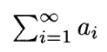# Positive Series: Definition, Examples, Convergence

A series is a positive series if all terms in the series are positive (an > 0). In other words:

a1 + a2 + ….

In summation notation, we can say that a seriesIs a positive series if ai≥ 0 for i = 1, 2, … (Eriksson et al., 2013).

## Partial Sums for Positive Series

The partial sums SN in a positive series always form an increasing sequence. In a sequence of partial sums, the next sum can be formed by adding a nonnegative number (Rogawski, 2007):To put this another way, the partial sums is a non-decreasing sequence. That’s because (Eriksson et al., 2013):## Dichotomy Theorem and Convergence for Positive Series

A positive series can behave in two ways (a “dichotomy”). The series either converges, or it diverges. The dichotomy theorem tells you whether the series converges or diverges, based on whether partial sums are bounded above (or not):

• If the partial sums are bounded above, the series converges.
• If the partial sums are not bounded above, the series diverges.

The dichotomy theorem isn’t the only way to tell if a positive series converges or diverges. You can use any of the following convergence tests: Integral Test, Comparison Test, Ratio Test or Root Test (Spinu, 2020). Not sure which series convergence test to choose? The following graphic (based on Professor Joe Kahlig’s original graphic) walks you through your choices.## References

Brand, L. (2013). Advanced Calculus. An Introduction to Classical Analysis. Dover Publications.
Eriksson, K. et al. (2013). Applied Mathematics: Body and Soul. Volume 2: Integrals and Geometry in IRn. Springer Berlin Heidelberg.
Kahlig, J. (2020). Choosing a Series Convergence Test. Retrieved July 29, 2020 from: https://www.math.tamu.edu/~kahlig/152wir/series-info.pdf
Rogawski, J. (2007). Calculus. W. H. Freeman.
Spinu, F. (2020). Convergence Tests for Positive Series. Retrieved July 29, 2020 from: http://math.jhu.edu/~fspinu/109_04_web/109/webnotes/convergence_tests.pdf

CITE THIS AS:
Stephanie Glen. "Positive Series: Definition, Examples, Convergence" From StatisticsHowTo.com: Elementary Statistics for the rest of us! https://www.statisticshowto.com/positive-series/